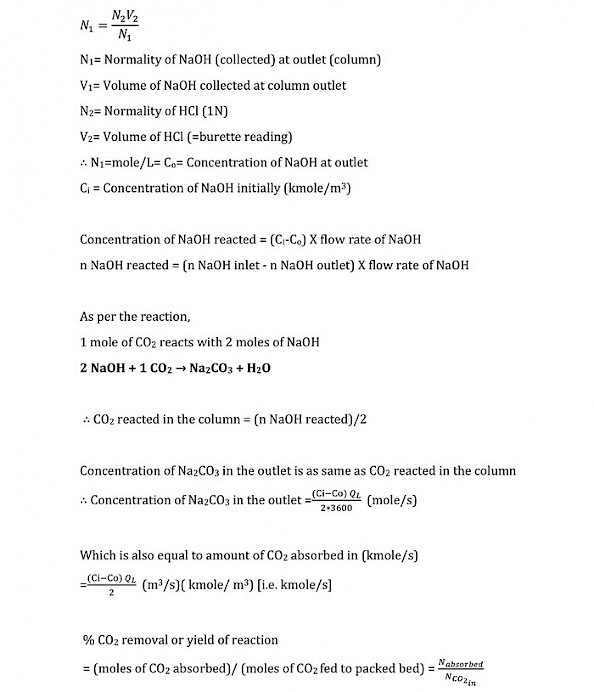#### Chemical Engineering Laboratory-II

Experiment 1 -  ABSORPTION IN PACKED BED

Objective: To study the absorption of carbon dioxide by aqueous sodium hydroxide solution in a packed bed column.

Aim:

1. To calculate the overall volumetric coefficient (KGa).

2. To calculate the number of transfer units (NTU).

3. To calculate the height of transfer units (HTU).

Observation & calculation:

Data given:

Atmospheric pressure P = 1 atm

Temperature=27°C

Diameter of the column dc = 0.048 m

Height of the column h = 0.750 m

Initial concentration of sodium hydroxide Ci = 2 mole/L  (2N)

Normality of HCl used for titration N2 = 1 g eq/L  (1N)

Molecular weight of CO2 MG = 44 g/mole

Molecular weight of air MA = 29 g/mole

Density of CO2 ρG at 0°C= 1.977 kg/m3 ; Density of CO2 ρG at 20°C= 1.8393 kg/m3

Density of air ρA at 0°C= 1.2922 kg/m3 ; Density of air ρA at 20°C = 1.2041 kg/m3

Observation table:

sl no

QL(LPH

QG(LPH)

QAir(LPH)

Volume of NaOH taken (ml)

1       10
2       10Reaction:

2NaOH + CO2 → Na2CO3 + H2O

Both NaOH and Na2CO3 react with HCl

NaOH  + HCl → NaCl + H2O

Na2CO3  + 2HCl → 2NaCl + H2O + CO2

BaCl2 + Na2CO3  → BaCO3  + 2NaCl

BaCl2 helps to separate the reacted NaOH from the unreacted NaOH through precipitation. During titration, the HCl reacts with unreacted NaOH so as to quantify how much CO2 was trapped by the hydroxide because the initial volume and molarity of NaOH is known. The end point of the titration process (i.e. from pink to colorless phase) marks the depletion of unreacted NaOH.

BaCl2 precipitates or solidifies the CO2 captured as BaCO3. After the addition of BaCl2, it is important to add phenolphthalein indicator in order to avoid CO2 adsorption from the atmosphere.

Calculations: